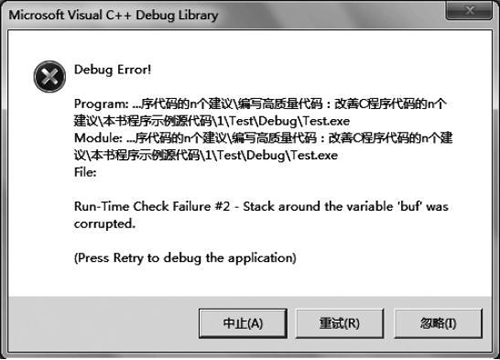## 位操作及其使用注意事项，C语言位操作及其使用方法详解

• 内容
• 评论
• 相关

C 语言中提供了 &（与）、|（或）、^（异或）、~（取反）、>>（右移）、<<（左移）6 种位操作符。我们可以在程序中合理地使用这些位操作符号来提高程序的运行效率，例如，对于下面的示例代码：

```int x=0;
int y=0;
x = 257 /8;
y = 456 % 32;```

```int x=0;
int y=0;
x = 257 >>3;
y = 456 - (456 >> 4 << 4);```

## 尽量避免对未知的有符号数执行位操作

```#include <stdio.h>
int main (void)
{
int x=0;
int y=0x80000000;
char buf[sizeof("128")];
x=sprintf(buf,"%u",y>>24);
if(x==-1||x>=sizeof(buf))
{
// 错误处理
}
printf(buf);
return 0;
}```0条评论